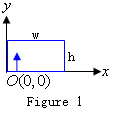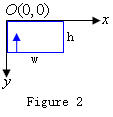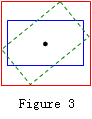Online JudgeProblem SetAuthorsOnline ContestsUser
Web Board
F.A.Qs
Statistical Charts
Problems
Submit Problem
Online Status
Prob.ID:
Register
Authors ranklist
Current Contest
Past Contests
Scheduled Contests
Award Contest
Register
Language:
Image Rotation
 Time Limit: 3000MS Memory Limit: 131072K Total Submissions: 128 Accepted: 38

Description

These days, Hunk is developing a special software for 2D digital image rotation. Here, the input image is described in the coordinate system shown in Figure 1 where the leftmost pixel of the image lies on the y-axis and the bottommost pixel of the image lies on the x-axis. The output image is described in the coordinate system shown in Figure 2 where and the leftmost pixel of the image lies on the y-axis, the topmost pixel lies on the x-axis.He considers the following:

1. Each image is a rectangle tiled with pixels. Pixels have discrete positions. The positions of their centers are referred to as their positions, which are usually denoted by order pairs of numbers (y, x).
2. In most cases, the dimensions of an image will change after rotation. As shown in Figure 3, rotating the original image (the blue rectangle) around its center will produce a new image (the red rectangle) with different height and width.3. Positions of pixels in the original image after rotation generally don't coincide with those of pixels in the new image. Rounding them to the nearest pixels might incur loss of image quality. An inverse transform method is used to tackle the difficulty. For each pixel in the new image, its position in the original image is computed using an inverse transform corresponding to the rotation to determine its color information.

The task is, for an image of h × w pixels and an angle α of counterclockwise rotation, to determine the dimensions in pixels of the rotated image, and for each pixel in the new image described in the coordinate system shown in Figure 2, to find its relative position in the original image described in the coordinate system shown in Figure 1.

Input

The input consists of multiple test cases.

Each test case consists of one line containing two positive integers h (1 ≤ h ≤ 128) and w (1 ≤ w ≤ 128) and a real number α (0 ≤ α ≤ 360) where h denotes the height of the image in pixels, w denotes the width of the image in pixels, α denotes the angle in degrees by which the image is rotated in counterclockwise direction.

Process to the end of input.

Output

For each test case, first output one line containing two integer H and W separated by one space meaning the new image has H × W pixels. Then output H lines each containing W coordinates representing the relative positions of pixels of the new image in the original image. If a position falls on the original image, output it in the format (y, x) with two decimal digits beyond the decimal point, otherwise output (−1.00, −1.00) instead. The coordinates are in the order in which pixels are aligned in the coordinate system shown in Figure 2. Output a blank line after each test case.

Sample Input

```3 3 90
3 4 60```

Sample Output

```3 3
(2.00,2.00) (1.00,2.00) (0.00,2.00)
(2.00,1.00) (1.00,1.00) (0.00,1.00)
(2.00,0.00) (1.00,0.00) (0.00,0.00)

4 4
(-1.00,-1.00) (-1.00,-1.00) (-1.00,-1.00) (-1.00,-1.00)
(-1.00,-1.00) (1.93,1.88) (1.07,2.38) (0.20,2.88)
(-1.00,-1.00) (1.43,1.02) (0.57,1.52) (-1.00,-1.00)
(-1.00,-1.00) (0.93,0.15) (0.07,0.65) (-1.00,-1.00)```

Hint

If the rotated image has dimensions lh × lw, then its dimensions in pixels are (floor(lh) + 1) × (floor(lw) + 1).

Make sure that your solution won’t output anything like “−0.00”.

Source

[Submit]   [Go Back]   [Status]   [Discuss]Home PageGo BackTo top